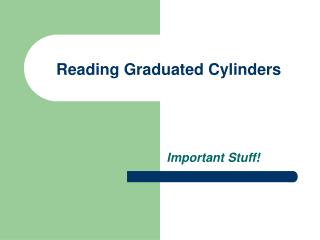DownloadDownload PresentationTélécharger la présentation- - - - - - - - - - - - - - - - - - - - - - - - - - - E N D - - - - - - - - - - - - - - - - - - - - - - - - - - -
##### Presentation Transcript

2. Graduated cylinders are used to measure the volume of liquid samples and are available in many different sizes.

3. Reading the Volume from a Graduated Cylinder • Determine the gradation markings on the GC. • Determine the volume contained in a graduated cylinder by reading the bottom of the meniscus at eye level. • Read the volume using all certain digits and one uncertain digit. • Certain digits are determined from the calibration marks on the cylinder. • The uncertain digit (the last digit of the reading) is estimated.

4. Depending on the size of the graduated cylinder and the graduations, the uncertain digit may be to the milliliter ( 1X ), the tenth of a milliliter ( 1.X ), or the hundredth of a milimeter ( 1.1X ). You will have to look at the graduated cylinder to determine how many digits to record!

5. Steps in brief… • Step 1: Determine the scale increment: • To find the scale increment, subtract the values of any two adjacent labeled graduations and divide by the number of intervals between them • Step 2: Use the graduations to find all certain digits: • Use the labeled graduations and the scale increment to find the certain digits in the measurement. • Step 3: Estimate the uncertain digit and obtain a reading: • Estimate the distance that the meniscus lies between the two graduations as a decimal fraction and multiply by the scale increment

6. Looking at a 10 mL cylinder • What is the volume you should record for the solution in the image to the right? • 6.31 mL correct • 6.3 incorrect • WHY?

7. Looking at a 25 mL cylinder • What is the volume you should record for the solution in the image to the right? • 21.50 mL correct • 21.5 incorrect • Why?

8. Looking at a 100 mL cylinder • What is the volume you should record for the solution in the image to the right? • 52.8 mL correct • 53 mL incorrect • Why?